# Volume + length - math problems

#### Number of problems found: 215

• MPG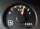252 miles a week fills up twice a week. Each fill is 38 gallons. What is the gas mileage of the car?
• Carpenter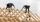From a wooden block carpenter cut off a small cuboid block with half the edge length. How many percent of wood did he cut off?
• Prism XThe prism with the edges of the lengths x cm, 2x cm, and 3x cm has volume 20250 cm3. What is the area of the surface of the prism?
• PrismThe base of the prism is a rhombus with a side 30 cm and a height 27 cm long. The height of the prism is 180% longer than the side length of the rhombus. Calculate the volume of the prism.
• Water tank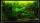The water tank shape cuboid has a width of 2.3 m and length twice larger. How high will reach water if water flows into 19 liters of water per second during 52 minutes?
• Car range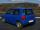Calculate the maximum range of car, if you can spend 10 euros, price of diesel is 1.55 Eur/l and car consumption is 3 l/100 km.
• PrismCalculate the volume of the rhombic prism. The prism base is a rhombus whose one diagonal is 47 cm, and the edge of the base is 28 cm. The edge length of the base of the prism and height is 3:5.
• Plastic pipeCalculate the plastic pipe's weight with diameter d = 70 mm and length 380 cm if the wall thickness is 4 mm and the density of plastic is 1367 kg/m3.
• Building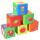Lenka has 22 cubes for the construction of building comprising three cubes in height, the width of two cubes, and the length of four cubes. Is she able to build an building with these cubes?Road embankment has a cross-section shape of an isosceles trapezoid with bases 5 m and 7 m, and 2 m long leg. How many cubic meters of soil is in embankment length of 1474 meters?
• TetrahedronCalculate height and volume of a regular tetrahedron whose edge has a length 6 cm.
• TerezaThe cube has an area of base 256 mm2. Calculate the edge length, volume, and area of its surface.
• PoolMr. Peter builds a pool shape of a four-sided prism with a rhombus base in the garden. Base edge length is 8 m, the distance of the opposite walls of the pool is 7 m. The estimated depth is 144 cm. How many hectoliters of water consume Mr. Peter to fill t
• Cu wireCopper wire has a length l = 820 m and diameter d = 10 mm. Calculate the weight if density of copper is ρ = 8500 kg/m3. Result round to one decimal place.
• PrismRight-angled prism, whose base is a right triangle with leg a = 3 cm and hypotenuse c = 13 cm, has the same volume as a cube with an edge length of 3 dm. a) Find the height of the prism b) Calculate the surface of the prism c) What percentage of the cube'
• ConeCircular cone of height 15 cm and volume 5699 cm3 is at one-third of the height (measured from the bottom) cut by a plane parallel to the base. Calculate the radius and circumference of the circular cut.
• Cube in a sphereThe cube is inscribed in a sphere with a volume 7253 cm3. Determine the length of the edges of a cube.
• CuboidCuboid with edge a=6 cm and space diagonal u=31 cm has volume V=900 cm3. Calculate the length of the other edges.
• PoolThe swimming pool is 10 m wide and 8 m long, and 153 cm deep. How many hectoliters of water are in it if the water is 30 cm below its upper edge?
• WaterInto a full cylindrical tank high 3 m with a base radius of 2.5 m, we insert cuboid with dimensions 1.7 m, 1.3 m, 1.9 m. How many liters of water will overflow out?

Do you have an exciting math question or word problem that you can't solve? Ask a question or post a math problem, and we can try to solve it.

We will send a solution to your e-mail address. Solved examples are also published here. Please enter the e-mail correctly and check whether you don't have a full mailbox.

Do you want to convert length units? Tip: Our volume units converter will help you with the conversion of volume units. Volume - math word problems. Length - math word problems.